# Electronics and Communication Engineering - Exam Questions Papers

16.

Consider the transmission line of length 37.5 cm, which is terminated into zero resistance. This line is being exited by a source of 1 GHz which has an internal impedance of 50 Ω. What is the input impedance of the line as seen by the source?

 A. 50 Ω B. Zero Ω C. 100 Ω D. Infinite Ω

Answer: Option D

Explanation:

No answer description available for this question. Let us discuss.

17.

The junction capacitance of a linearly graded pn junction (with applied voltage = VB) is proportional to

 A.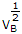B.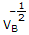C.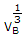D.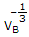Answer: Option D

Explanation:

No answer description available for this question. Let us discuss.

18.

Consider the following statements:
For a square waveguide of cross-section 3m x 3m it has been found

1. At 6 GHz dominant mode will propagate
2. At 4 GHz only dominant modes and evanescent
3. At 11 GHz all the modes and no higher order mode will propagate
4. At 7 GHz degenerate modes will propagate
Which of the above statements are correct?

 A. 1 and 2 only B. 1, 2 and 4 C. 2 and 3 only D. 2, 3 and 4

Answer: Option A

Explanation:

No answer description available for this question. Let us discuss.

19.

The circuit shown depicts :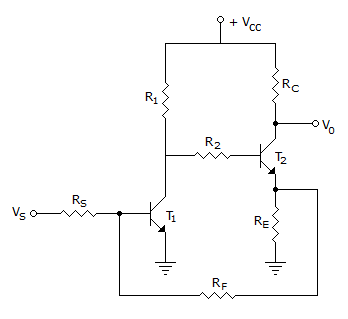A. voltage series negative feedback B. voltage shunt negative feedback C. current series negative feedback D. current shunt negative feedback

Answer: Option D

Explanation:

No answer description available for this question. Let us discuss.

20.

Which of the transistor models is most preferred for the analysis of a transistor circuit both at mid-band and at high frequencies?

 A. h-parameter model B. y-parameter model C. s-parameter model D. hybrid-p model

Answer: Option D

Explanation:

No answer description available for this question. Let us discuss.

#### Current Affairs 2021

Interview Questions and Answers